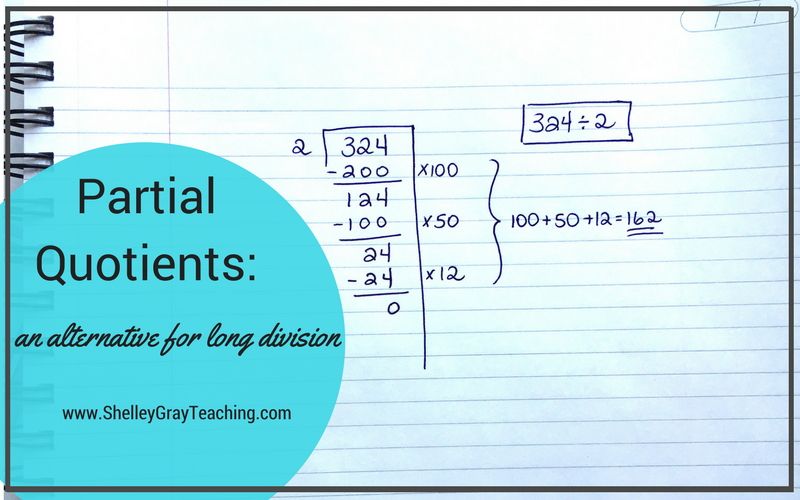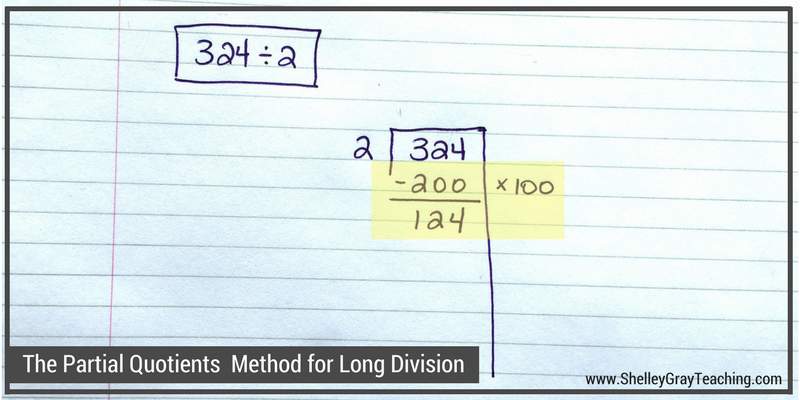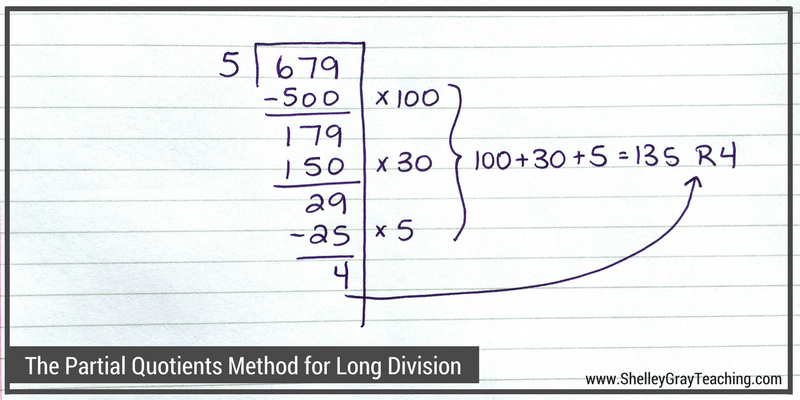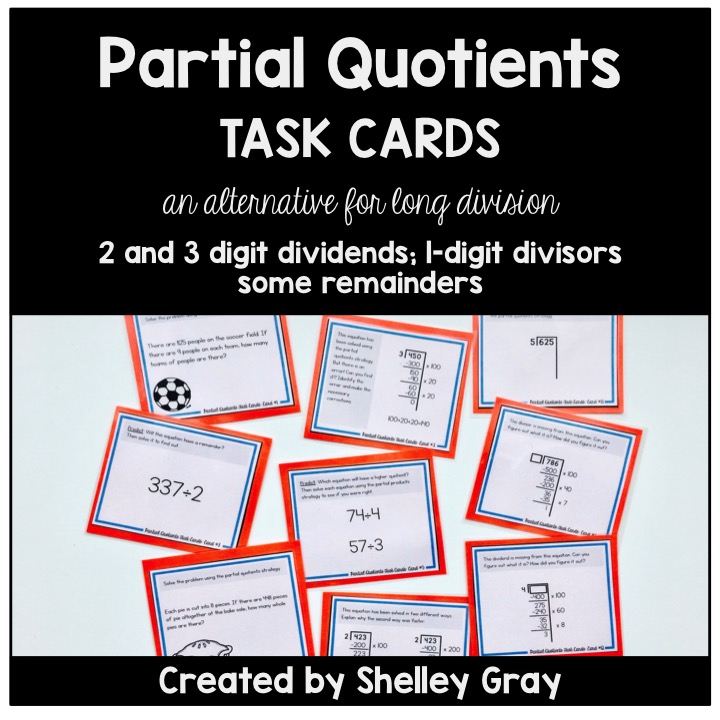# Partial Quotients: an alternative for traditional long divisionLong division is often considered one of the most challenging topics to teach. Luckily, there are strategies that we can teach to make multi-digit division easier to understand and perform.

The Partial Quotients method is one of these strategies. It is a mental math based approach that will enhance number sense understanding. Students solve the equation by subtracting multiples until they get down to 0, or as close to 0 as possible.

If you would like to try this strategy in your classroom, you may want to start with the Box Method/Area Model, which you can read more about HERE. The Box Method uses the same approach as Partial Quotients, but is organized differently and works well as an introduction.

Let’s learn how to perform the Partial Quotients strategy for long division!

Below, I have included both a video tutorial and step-by-step instructions.

VIDEO TUTORIAL

STEP-BY-STEP INSTRUCTIONS

Suppose that we want to solve the equation 324÷2.

STEP 1:
Write the equation as shown below.STEP 2:
Now it is time to start figuring out how many groups of 2 will fit into 324. We want to use numbers that are easy to work with, so we try to use friendly numbers like 10, 100, etc. We know that at least 100 groups of 2 fit into 324, so let’s start with that. We write x100 on the right side to symbolize that we are taking out 100 groups. 100 groups of 2 makes 200, so we subtract 200 from 324. This leaves us with 124.STEP 3:
Now we need to figure out how many more groups of 2 we can make from 124. We know that we can make at least 50 more groups. We write x50 on the right side to symbolize the 50 groups of 2. 50 groups of 2 makes 100, so we take 100 away from 124 and we are left with 24.STEP 4:
How many more groups of 2 can we make from 24? We know that we can make 12 more groups, so we write x12 on the right side. 12 groups of 2 makes 24, so we take away 24. We are left with 0, which means that we do not have a remainder.STEP 4:
To find our quotient, we add up the three partial quotients. In this case, we add 100+50+12 to make 162. So 324÷2=162.Here’s one more example. Let’s solve 679÷5:We know that there are at least 100 groups of 5 in 679, so we wrote x100. We then subtract 100 groups of 5, or 500, from the 679, and we are left with 179.

We know that there are at least 30 more groups of 5 in 179. 30 groups of 5 makes 150, so we subtract 150 from 179 and are left with 29.

We know that there are at least 5 more groups of 5 in 29. 5 groups of 5 makes 25, so we subtract 25 from 29 and are left with 4.

We cannot make any more groups of 5 out of 4, so 4 is our remainder.

To find our quotient, we add the partial quotients (100+30+5) plus the remainder 4, to make 135 R4.

HELPFUL RESOURCES FOR THE PARTIAL QUOTIENTS STRATEGY FOR DIVISION

I would love to help you teach the partial quotients strategy for long division in your classroom. You may find the following resources helpful:

FREE MINI COURSE

REGISTER HERE for a free mini course – Teaching Multi-Digit Multiplication and Division for REAL Understanding. It will only take you about an hour to complete and you’ll leave with a ton of new ideas, free resources, a PD Certificate, and more!These task cards give students the opportunity to practice the partial quotients strategy for long division in a variety of different ways. Students will calculate quotients, solve division problems, figure out missing dividends and divisors, think about how to efficiently solve an equation using the partial quotients strategy, and more. See the Partial Quotients Task Cards HERE or the Big Bundle of Long Division Task Cards HERE.THE LONG DIVISION STATION

The Long Division Station is a self-paced, student-centered math station for long division. Students gradually learn a variety of strategies for long division, the partial quotients strategy being one of them. One of the greatest advantages to this Math Station is that is allows you to target every student and their unique abilities so that everyone is appropriately challenged. See The Long Division Station HERE.OR SEE ALL RESOURCES HERE.